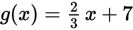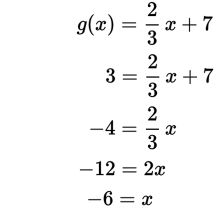# SAT Math Multiple Choice Question 500: Answer and Explanation

### Test Information

Question: 500

5. Given the function, what domain value corresponds to a range value of 3?

• A. -6
• B. -2
• C. 6
• D. 9

Explanation:

A

Difficulty: Medium

Category: Passport to Advanced Math / Functions

Strategic Advice: Don't answer this question too quickly—you may be tempted to substitute 3 for x, but 3 is the output (range), not the input (domain).

Getting to the Answer: The given range value is an output value, so substitute 3 for g(x) and use inverse operations to solve for x, which is the corresponding domain value.You could also graph the function and find the value of x (the domain value) for which the value of y (the range value) is 3. The point on the graph is (-6, 3).